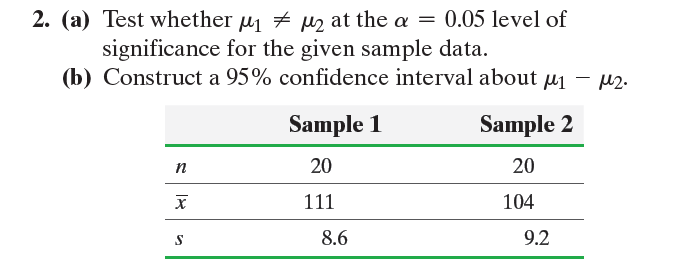×
Get Full Access to Statistics: Informed Decisions Using Data - 5 Edition - Chapter 11.3 - Problem 2
Get Full Access to Statistics: Informed Decisions Using Data - 5 Edition - Chapter 11.3 - Problem 2

×ISBN: 9780134133539 240

## Solution for problem 2 Chapter 11.3

Statistics: Informed Decisions Using Data | 5th Edition

• Textbook Solutions
• 2901 Step-by-step solutions solved by professors and subject experts
• Get 24/7 help from StudySoup virtual teaching assistantsStatistics: Informed Decisions Using Data | 5th Edition

4 5 1 295 Reviews
12
0
Problem 2

In Problems 1–6, assume that the populations are normally distributed.

2. (a) Test whether m1 ≠ m2 at the a = 0.05 level of significance for the given sample data.

(b) Construct a 95% confidence interval about m1 - m2.Step-by-Step Solution:
Step 1 of 3

Lecture 3 Nicole Rubenstein September 5, 2017 3 Probability Properties (2.4-2.5, 2.7-2.9) De▯nition 3.1. An experiment is an activity that involves chance that leads to results, which can be repeated. De▯nition 3.2. A sample point is a possible result of a single trial of an experiment. De▯nition 3.3. An event is one or more possible outcomes of an experiment (i.e. a collection of sample points). The probability of an event A occurring is denoted P(A). There are two di▯erent types of events: 1. Simple event: only one sample point 2. Compound event: more than one sample point De▯nition 3.4. A sample space associated with an experiment is the set of all possible sample points, usually denoted by S. Example 3.1. Consider ipping a coin once. ▯ Sample points: H (heads up), T (tails up) ▯ Sample space: S = fH;Tg Example 3.2. Consider rolling a fair 6-sided die. ▯ Sample space: S = f1;2;3;4;5;6g ▯ A simple event: fyou roll a 1g = f1g ▯ A compound event: fan even number is rolled = f2;4;6g De▯nition 3.5. The compliment of an event A (or the negation of the description of the event), denoted by [A] or A , is the event that A does not occur. Example 3.3. The compliment of fWe roll an even numberg is: c f2;4;6g = f1;3;5g: De▯nition 3.6. The union of two events is the event that either of those two events occurs. Example 3.4. fWe roll an even numberg [ fWe roll a num

Step 2 of 3

Step 3 of 3

## Discover and learn what students are asking

Statistics: Informed Decisions Using Data : Estimating a Population Mean
?The procedure for constructing a t-interval is robust. Explain what this means.

Unlock Textbook Solution